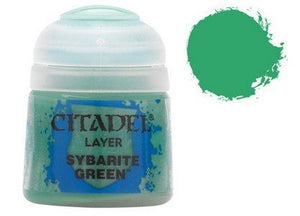# Citadel Layer 1: Sybarite Green

Games Workshop

• \$14.73
Unit price per
• Save \$12.74

• C

• i

• t

• a

• d

• e

• l

• L

• a

• y

• e

• r

• p

• a

• i

• n

• t

• s

• a

• r

• e

• h

• i

• g

• h

• q

• u

• a

• l

• i

• t

• y

• a

• c

• r

• y

• l

• i

• c

• p

• a

• i

• n

• t

• s

• ,

• a

• n

• d

• w

• i

• t

• h

• 7

• 0

• o

• f

• t

• h

• e

• m

• i

• n

• t

• h

• e

• C

• i

• t

• a

• d

• e

• l

• P

• a

• i

• n

• t

• r

• a

• n

• g

• e

• ,

• y

• o

• u

• h

• a

• v

• e

• a

• h

• u

• g

• e

• r

• a

• n

• g

• e

• o

• f

• c

• o

• l

• o

• u

• r

• s

• a

• n

• d

• t

• o

• n

• e

• s

• t

• o

• c

• h

• o

• o

• s

• e

• f

• r

• o

• m

• w

• h

• e

• n

• y

• o

• u

• p

• a

• i

• n

• t

• y

• o

• u

• r

• m

• i

• n

• i

• a

• t

• u

• r

• e

• s

• .

Sybarite Green is a Citadel Layer paint. Citadel Layer paints are high quality acrylic paints, and with 70 of them in the Citadel Paint range, you have a huge range of colours and tones to choose from when you paint your miniatures. They are designed to be used straight over Citadel Base paints (and each other) without any mixing. By using several layers you can create a rich, natural finish on your models that looks fantastic on the battlefield. This pot contains 12ml of Sybarite Green, one of 70 Layer paints in the Citadel Paint range. As with all of our paints, it is a non-toxic, water-based acrylic paint designed for use on plastic, metal, and resin Citadel miniatures.